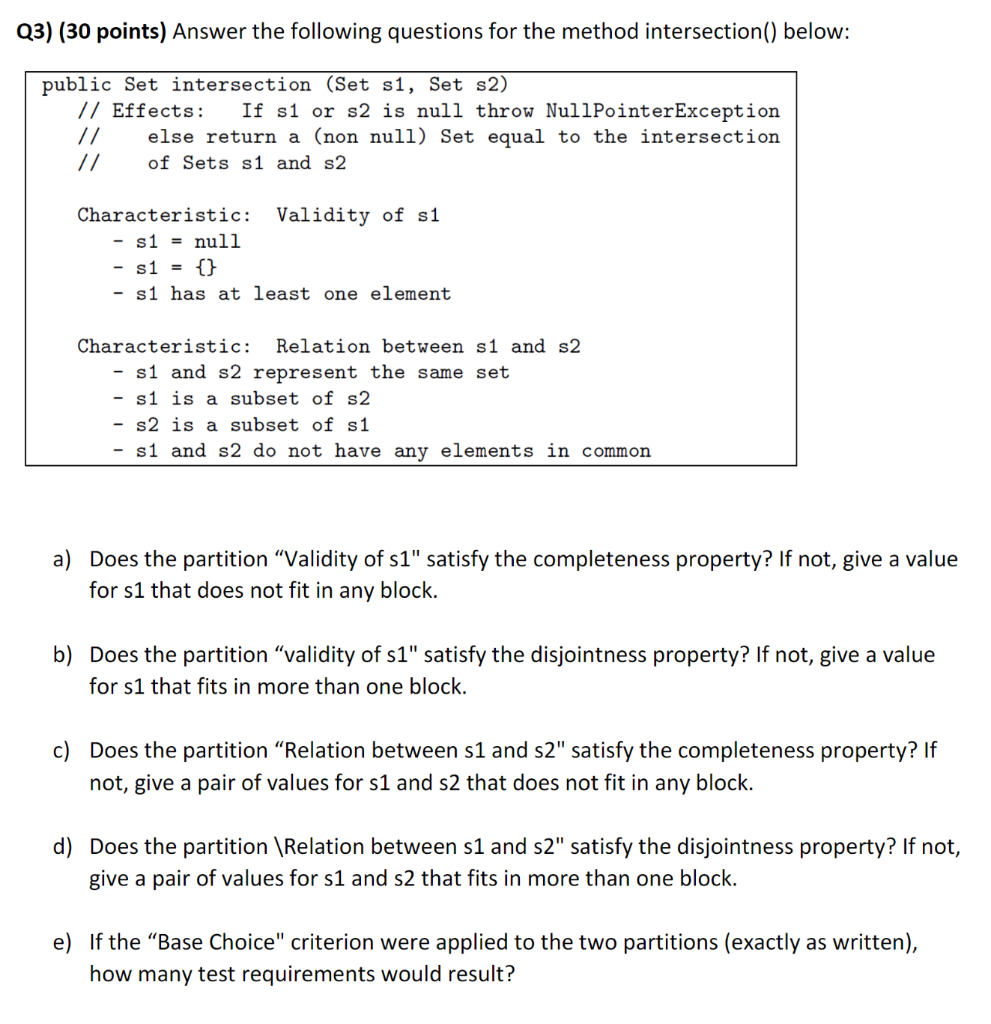# Question Only have to do D, E Can you explain clearly Q3) (30 points) Answer the following questions for the method intersection() below: public Set intersection (Set s1, Set s2) // Effects: If s1 or s2 is null throw NullPointerException // else return a (non null) Set equal to the intersection // of Sets s1 and s2 Characteristic: Validity of s1 - s1 = null s1 = {} - s1 has at least one element Characteristic: Relation between s1 and s2 - s1 and s2 represent the same set s1 is a subset of s2 s2 is a subset of s1 - s1 and s2 do not have any elements in common a) Does the partition “Validity of s1" satisfy the completeness property? If not, give a value for s1 that does not fit in any block. b) Does the partition “validity of s1" satisfy the disjointness property? If not, give a value for s1 that fits in more than one block. c) Does the partition “Relation between s1 and 52" satisfy the completeness property? If not, give a pair of values for s1 and s2 that does not fit in any block. d) Does the partition \Relation between s1 and s2" satisfy the disjointness property? If not, give a pair of values for s1 and s2 that fits in more than one block. e) If the “Base Choice" criterion were applied to the two partitions (exactly as written), how many test requirements would result?PUZXHQ The Asker · Computer Science

Only have to do D, E
Can you explain clearlyTranscribed Image Text: Q3) (30 points) Answer the following questions for the method intersection() below: public Set intersection (Set s1, Set s2) // Effects: If s1 or s2 is null throw NullPointerException // else return a (non null) Set equal to the intersection // of Sets s1 and s2 Characteristic: Validity of s1 - s1 = null s1 = {} - s1 has at least one element Characteristic: Relation between s1 and s2 - s1 and s2 represent the same set s1 is a subset of s2 s2 is a subset of s1 - s1 and s2 do not have any elements in common a) Does the partition “Validity of s1" satisfy the completeness property? If not, give a value for s1 that does not fit in any block. b) Does the partition “validity of s1" satisfy the disjointness property? If not, give a value for s1 that fits in more than one block. c) Does the partition “Relation between s1 and 52" satisfy the completeness property? If not, give a pair of values for s1 and s2 that does not fit in any block. d) Does the partition \Relation between s1 and s2" satisfy the disjointness property? If not, give a pair of values for s1 and s2 that fits in more than one block. e) If the “Base Choice" criterion were applied to the two partitions (exactly as written), how many test requirements would result?
More
Transcribed Image Text: Q3) (30 points) Answer the following questions for the method intersection() below: public Set intersection (Set s1, Set s2) // Effects: If s1 or s2 is null throw NullPointerException // else return a (non null) Set equal to the intersection // of Sets s1 and s2 Characteristic: Validity of s1 - s1 = null s1 = {} - s1 has at least one element Characteristic: Relation between s1 and s2 - s1 and s2 represent the same set s1 is a subset of s2 s2 is a subset of s1 - s1 and s2 do not have any elements in common a) Does the partition “Validity of s1" satisfy the completeness property? If not, give a value for s1 that does not fit in any block. b) Does the partition “validity of s1" satisfy the disjointness property? If not, give a value for s1 that fits in more than one block. c) Does the partition “Relation between s1 and 52" satisfy the completeness property? If not, give a pair of values for s1 and s2 that does not fit in any block. d) Does the partition \Relation between s1 and s2" satisfy the disjointness property? If not, give a pair of values for s1 and s2 that fits in more than one block. e) If the “Base Choice" criterion were applied to the two partitions (exactly as written), how many test requirements would result?